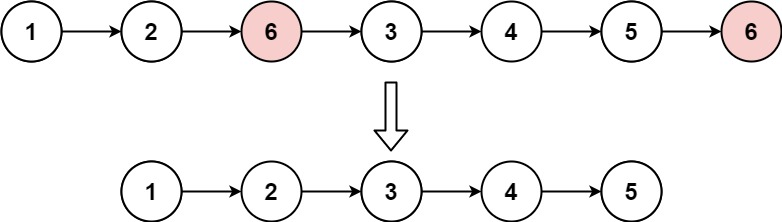# GeetCode Hub

Given the `head` of a linked list and an integer `val`, remove all the nodes of the linked list that has `Node.val == val`, and return the new head.

Example 1:```Input: head = [1,2,6,3,4,5,6], val = 6
Output: [1,2,3,4,5]
```

Example 2:

```Input: head = [], val = 1
Output: []
```

Example 3:

```Input: head = [7,7,7,7], val = 7
Output: []
```

Constraints:

• The number of nodes in the list is in the range `[0, 104]`.
• `1 <= Node.val <= 50`
• `0 <= val <= 50`

/** * Definition for singly-linked list. * public class ListNode { * int val; * ListNode next; * ListNode() {} * ListNode(int val) { this.val = val; } * ListNode(int val, ListNode next) { this.val = val; this.next = next; } * } */ class Solution { public ListNode removeElements(ListNode head, int val) { } }